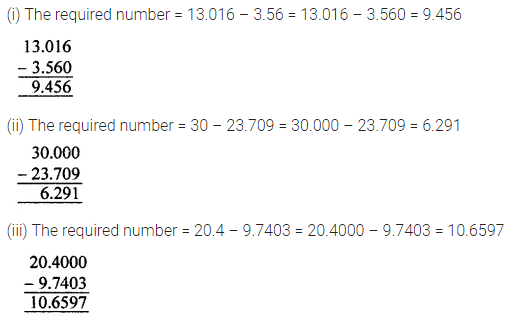# ML Aggarwal Class 7 Solutions for ICSE Maths Chapter 2 Fractions and Decimals Ex 2.5

## ML Aggarwal Class 7 Solutions for ICSE Maths Chapter 2 Fractions and Decimals Ex 2.5

Question 1.
Write the following numbers in the expanded form:
(i) 20.03
(ii) 200.03
(iii) 2.034
Solution: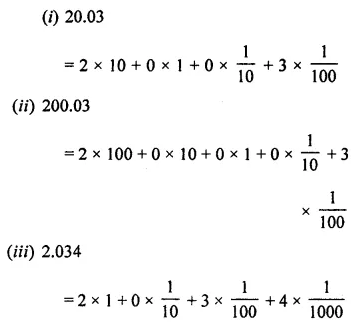Question 2.
Write the place value of digit 2 in the following decimal numbers:
(i) 2.56
(ii) 21.37
(iii) 10.25
(iv) 63.352
Solution: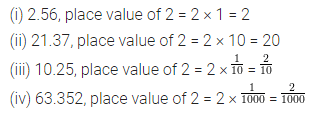Question 3.
Convert the following decimal numbers to frac ions (in simplest form):
(i) 0.8
(ii) 0.225
(iii) 0. 0092
(iv) 3.025
Solution: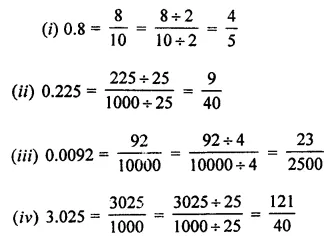Question 4.
Convert the following decimals to mixed fractions:
(i) 5.05
(ii) 63.125
(iii) 17.075
(iv) 317.0006
Solution: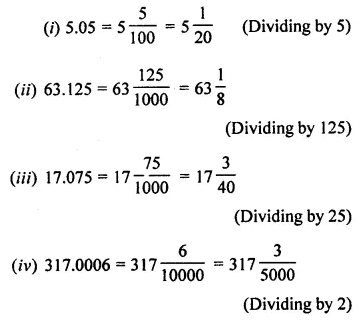Question 5.
Convert the following fractions into decimal numbers:
(i) $$\frac { 3 }{ 5 }$$
(ii) $$\frac { 7 }{ 8 }$$
(iii) 3$$\frac { 5 }{ 16 }$$
(iv) 137$$\frac { 13 }{ 62 }$$
Solution:Question 6.
Which is greater?
(i) 0.5 or 0.05
(ii) 7 or 0.7
(iii) 2.03 or 2.30
(iv) 0.8 or 0.88
Solution: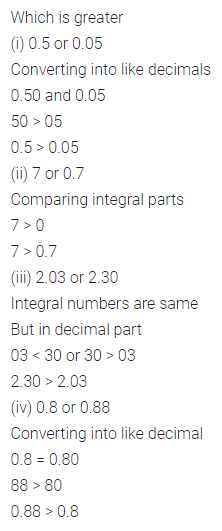Question 7.
Arrange the following decimal numbers in ascending order:
(i) 38.02, 38.021, 3.802, 83.02, 38.002
(ii) 46.542, 46.452, 46.254, 46.05, 64.542, 46.0542
Solution: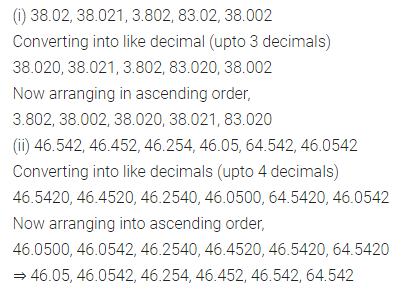Question 8.
Arrange the following decimal numbers in descending order:
(i) 5.6, 0.93, 1.87, 1.9, 1.78, 0.39
(ii) 71.201, 20.1, 2.01, 3.1, 2.14, 0.652
Solution: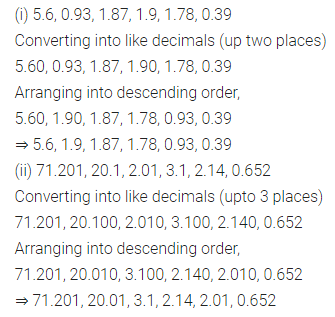Question 9.
Express as rupees using decimals:
(i) 7 paise
(ii) 77 rupees 77 paise
(iii) 235 paise
Solution:Question 10.
Express 5 cm in metre and kilometre.
Solution: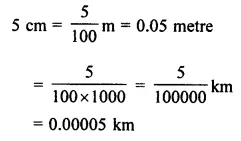Question 11.
Express in kg using decimals:
(i) 200 g
(ii) 3470 g
(iii) 4 kg 8 g
Solution: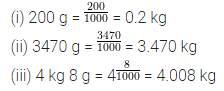Question 12.
(i) 5.765, 9.2, 3.08
(ii) 15.49, 8.3572, 0.903, 7.8
Solution:Question 13.
Workout the following:
(i) 72.53 – 46.782
(ii) 18.376 – 5.43 – 8.8976
(iii) 28.5 – 9.708 – 6.234
(iv) 8.2 – 4.56 – 0.7912 + 2.67
Solution: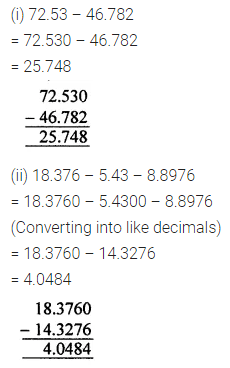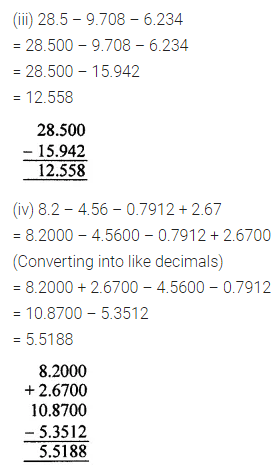Question 14.
(i) What number added to 3.56 gives 13.016?
(ii) What number should be subtracted from 30 to get 23.709?
(iii) What is the excess of 20.4 over 9.7403?
Solution: